Publicité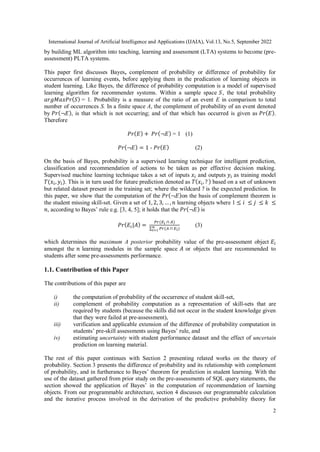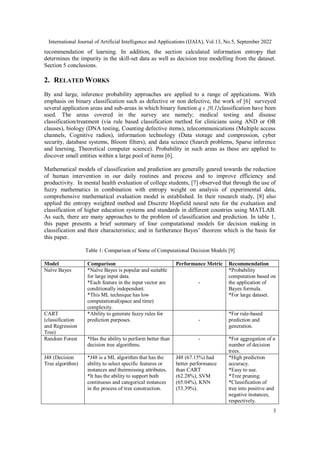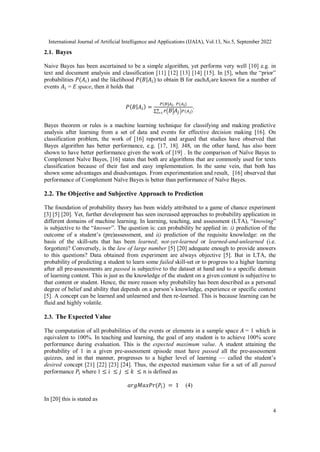Publicité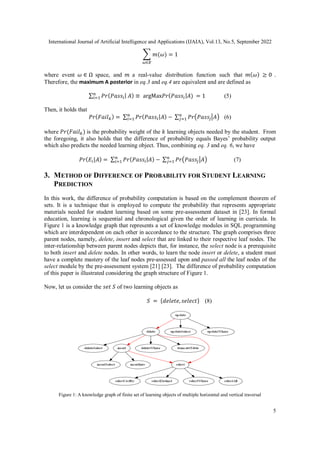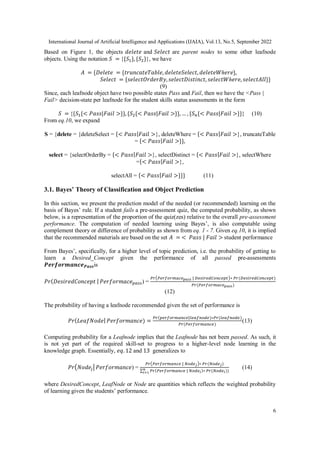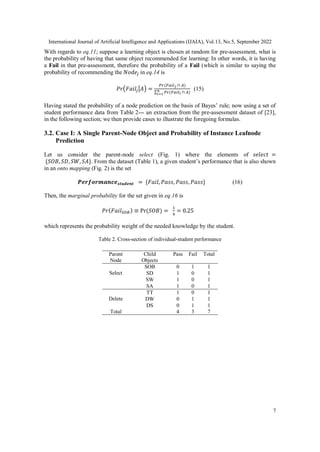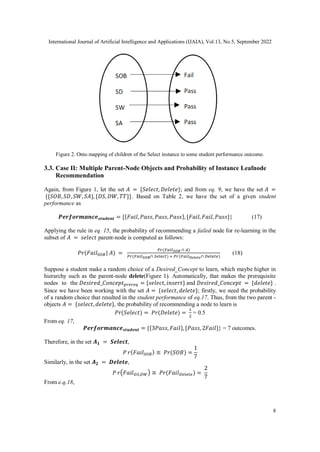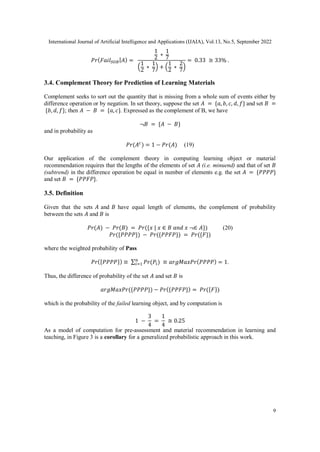Publicité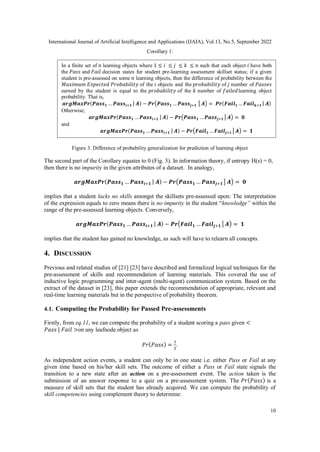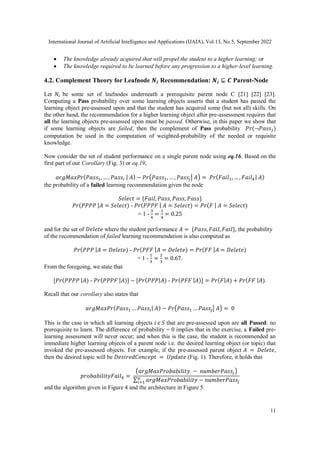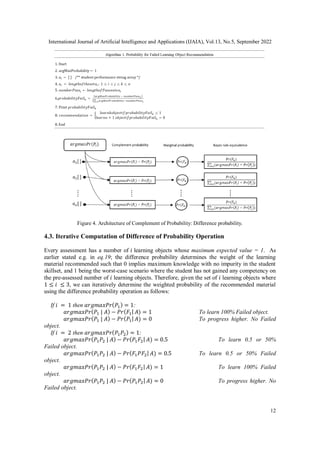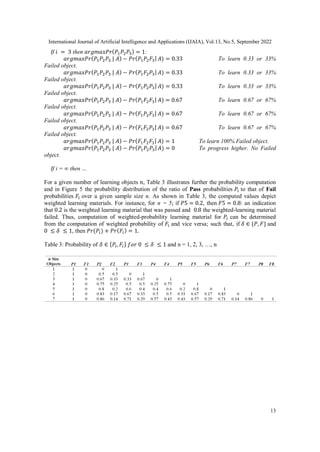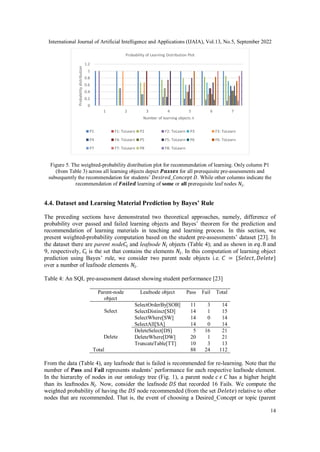Publicité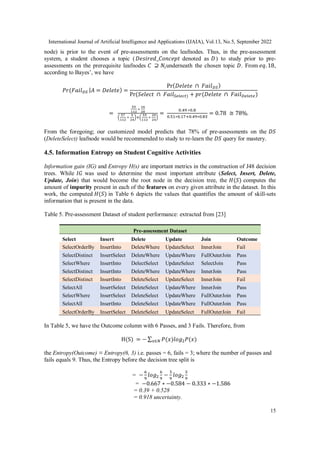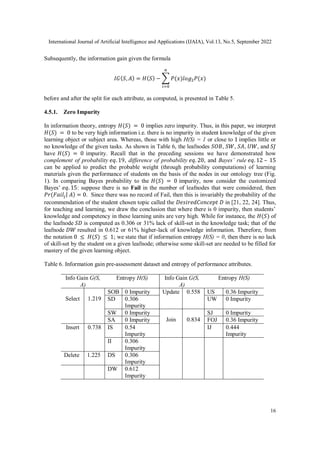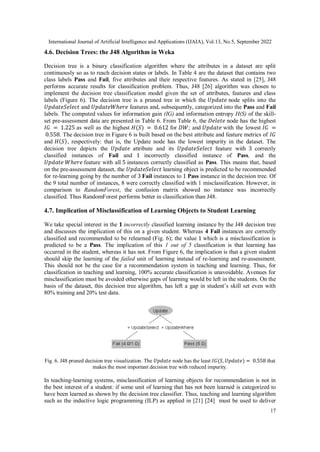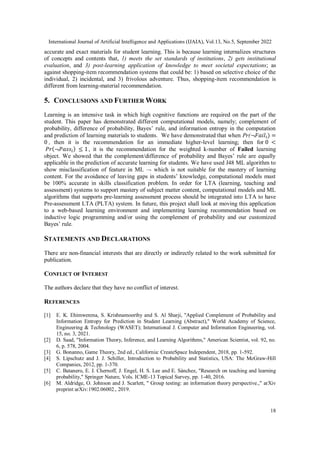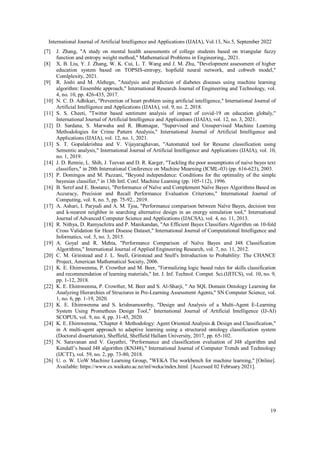Prochain SlideShare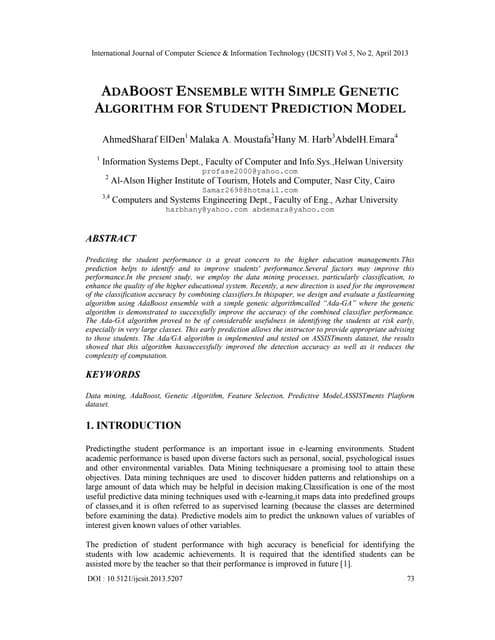ADABOOST ENSEMBLE WITH SIMPLE GENETIC ALGORITHM FOR STUDENT PREDICTION MODEL
Chargement dans ... 3
1 sur 19
Publicité

### DIFFERENCE OF PROBABILITY AND INFORMATION ENTROPY FOR SKILLS CLASSIFICATION AND PREDICTION IN STUDENT LEARNING

1. International Journal of Artificial Intelligence and Applications (IJAIA), Vol.13, No.5, September 2022 DOI: 10.5121/ijaia.2022.13501 1 DIFFERENCE OF PROBABILITY AND INFORMATION ENTROPY FOR SKILLS CLASSIFICATION AND PREDICTION IN STUDENT LEARNING Kennedy Efosa Ehimwenma1 , Safiya Al Sharji2 and Maruf Raheem3 1 Department of Computer Science, College of Science and Technology, Wenzhou-Kean University, China 2 Department of Information Technology, University of Technology and Applied Sciences, Muscat, Oman 3 Department of Statistics University of Uyo, Nigeria ABSTRACT The probability of an event is in the range of [0, 1]. In a sample space S, the value of probability determines whether an outcome is true or false. The probability of an event Pr(A) that will never occur = 0. The probability of the event Pr(B) that will certainly occur = 1. This makes both events A and B thus a certainty. Furthermore, the sum of probabilities Pr(E1) + Pr(E2) + … + Pr(En) of a finite set of events in a given sample space S = 1. Conversely, the difference of the sum of two probabilities that will certainly occur is 0. Firstly, this paper discusses Bayes’ theorem, then complement of probability and the difference of probability for occurrences of learning-events, before applying these in the prediction of learning objects in student learning. Given the sum total of 1; to make recommendation for student learning, this paper submits that the difference of argMaxPr(S) and probability of student-performance quantifies the weight of learning objects for students. Using a dataset of skill-set, the computational procedure demonstrates: i) the probability of skill-set events that has occurred that would lead to higher level learning; ii) the probability of the events that has not occurred that requires subject-matter relearning; iii) accuracy of decision tree in the prediction of student performance into class labels; and iv) information entropy about skill-set data and its implication on student cognitive performance and recommendation of learning . KEYWORDS Complement of probability, Bayes’ rule, computational education, pre-learning assessment, information theory, SQL ontology 1. INTRODUCTION Indeed, the theory of probability application is far beyond determining the outcome of a rolled dice, picking a colored ball from an urn or a card from a set in a deck of cards . Probability is thus a veritable method for drawing inferences i.e. arriving at conclusions from given evidence and reasoning in a given domain problem. While approaches such as deductive and inductive logic are based on logical reasoning from available evidence, approaches by probabilistic methods are largely dependent on statistical computation of facts and the evidence provided. Assessment is an indispensable part of teaching and learning process. For subject-matter mastery, this paper argues using an adaptable predictive algorithmic model that pre-learning assessment which unravels and determines a student’s state of knowledge before teaching and further learning should be an integral part of teaching and learning systems. That, this should be achieve
2. International Journal of Artificial Intelligence and Applications (IJAIA), Vol.13, No.5, September 2022 2 by building ML algorithm into teaching, learning and assessment (LTA) systems to become (pre- assessment) PLTA systems. This paper first discusses Bayes, complement of probability or difference of probability for occurrences of learning events, before applying them in the predication of learning objects in student learning. Like Bayes, the difference of probability computation is a model of supervised learning algorithm for recommender systems. Within a sample space 𝑆, the total probability 𝑎𝑟𝑔𝑀𝑎𝑥𝑃𝑟(𝑆) = 1. Probability is a measure of the ratio of an event E in comparison to total number of occurrences S. In a finite space A, the complement of probability of an event denoted by 𝑃𝑟(¬𝐸), is that which is not occurring; and of that which has occurred is given as 𝑃𝑟(𝐸). Therefore 𝑃𝑟(𝐸) + 𝑃𝑟(¬𝐸) = 1 (1) 𝑃𝑟(¬𝐸) = 1 - 𝑃𝑟(𝐸) (2) On the basis of Bayes, probability is a supervised learning technique for intelligent prediction, classification and recommendation of actions to be taken as per effective decision making. Supervised machine learning technique takes a set of inputs 𝑥𝑖 and outputs 𝑦𝑖 as training model 𝑇(𝑥𝑖, 𝑦𝑖). This is in turn used for future prediction denoted as 𝑇(𝑥𝑖, ? ) based on a set of unknown but related dataset present in the training set; where the wildcard ? is the expected prediction. In this paper, we show that the computation of the 𝑃𝑟(¬𝐸)on the basis of complement theorem is the student missing skill-set. Given a set of 1, 2, 3, … , 𝑛 learning objects where 1 ≤ 𝑖 ≤ 𝑗 ≤ 𝑘 ≤ 𝑛, according to Bayes’ rule e.g. [3, 4, 5]; it holds that the 𝑃𝑟(¬𝐸) is 𝑃𝑟(𝐸𝑖|𝐴) = 𝑃𝑟(𝐸𝑖 ∩ 𝐴) ∑ 𝑃𝑟(𝐴 ∩ 𝐸𝑖) 𝑛 𝑖=1 (3) which determines the maximum A posterior probability value of the pre-assessment object 𝐸𝑖 amongst the 𝑛 learning modules in the sample space 𝐴 or objects that are recommended to students after some pre-assessments performance. 1.1. Contribution of this Paper The contributions of this paper are i) the computation of probability of the occurrence of student skill-set, ii) complement of probability computation as a representation of skill-sets that are required by students (because the skills did not occur in the student knowledge given that they were failed at pre-assessment), iii) verification and applicable extension of the difference of probability computation in students’ pre-skill assessments using Bayes’ rule, and iv) estimating uncertainty with student performance dataset and the effect of uncertain prediction on learning material. The rest of this paper continues with Section 2 presenting related works on the theory of probability. Section 3 presents the difference of probability and its relationship with complement of probability, and in furtherance to Bayes’ theorem for prediction in student learning. With the use of the dataset gathered from prior study on the pre-assessments of SQL query statements, the section showed the application of Bayes’ in the computation of recommendation of learning objects. From our programmable architecture, section 4 discusses our programmable calculation and the iterative process involved in the derivation of the predictive probability theory for
3. International Journal of Artificial Intelligence and Applications (IJAIA), Vol.13, No.5, September 2022 3 recommendation of learning. In addition, the section calculated information entropy that determines the impurity in the skill-set data as well as decision tree modelling from the dataset. Section 5 conclusions. 2. RELATED WORKS By and large, inference probability approaches are applied to a range of applications. With emphasis on binary classification such as defective or non defective, the work of  surveyed several application areas and sub-areas in which binary function q ϵ {0,1}classification have been used. The areas covered in the survey are namely; medical testing and disease classification/treatment (via rule based classification method for clinicians using AND or OR clauses), biology (DNA testing, Counting defective items), telecommunications (Multiple access channels, Cognitive radios), information technology (Data storage and compression, cyber security, database systems, Bloom filters), and data science (Search problems, Sparse inference and learning, Theoretical computer science). Probability in such areas as these are applied to discover small entities within a large pool of items . Mathematical models of classification and prediction are generally geared towards the reduction of human intervention in our daily routines and process and to improve efficiency and productivity. In mental health evaluation of college students,  observed that through the use of fuzzy mathematics in combination with entropy weight on analysis of experimental data, comprehensive mathematical evaluation model is established. In their research study,  also applied the entropy weighted method and Discrete Hopfield neural nets for the evaluation and classification of higher education systems and standards in different countries using MATLAB. As such, there are many approaches to the problem of classification and prediction. In table 1, this paper presents a brief summary of four computational models for decision making in classification and their characteristics; and in furtherance Bayes’ theorem which is the basis for this paper. Table 1: Comparison of Some of Computational Decision Models  Model Comparison Performance Metric Recommendation Naïve Bayes *Naïve Bayes is popular and suitable for large input data. *Each feature in the input vector are conditionally independent. *This ML technique has low computational(space and time) complexity. - *Probability computation based on the application of Bayes formula. *For large dataset. CART (classification and Regression Tree) *Ability to generate fuzzy rules for prediction purposes. - *For rule-based prediction and generation. Random Forest *Has the ability to perform better than decision tree algorithms. - *For aggregation of n number of decision trees. J48 (Decision Tree algorithm) *J48 is a ML algorithm that has the ability to select specific features or instances and theirmissing attributes. *It has the ability to support both continuous and categorical instances in the process of tree construction. J48 (67.15%) had better performance than CART (62.28%), SVM (65.04%), KNN (53.39%). *High prediction accuracy. *Easy to use. *Tree pruning. *Classification of tree into positive and negative instances, respectively.
4. International Journal of Artificial Intelligence and Applications (IJAIA), Vol.13, No.5, September 2022 4 2.1. Bayes Naive Bayes has been ascertained to be a simple algorithm, yet performs very well  e.g. in text and document analysis and classification     . In , when the “prior” probabilities 𝑃(𝐴𝑖) and the likelihood 𝑃(𝐵|𝐴𝑖) to obtain B for each𝐴𝑖are known for a number of events 𝐴𝑖 = E space, then it holds that 𝑃(𝐵|𝐴𝑖) = 𝑃(𝐵|𝐴𝑖. 𝑃(𝐴𝑖) ∑ 𝑃(𝐵|𝐴𝑗)𝑃(𝐴𝑗) 𝑛 𝑖=1 . Bayes theorem or rules is a machine learning technique for classifying and making predictive analysis after learning from a set of data and events for effective decision making . On classification problem, the work of  reported and argued that studies have observed that Bayes algorithm has better performance, e.g. [17, 18]. J48, on the other hand, has also been shown to have better performance given the work of  . In the comparison of Naïve Bayes to Complement Naïve Bayes,  states that both are algorithms that are commonly used for texts classification because of their fast and easy implementation. In the same vein, that both has shown some advantages and disadvantages. From experimentation and result,  observed that performance of Complement Naïve Bayes is better than performance of Naïve Bayes. 2.2. The Objective and Subjective Approach to Prediction The foundation of probability theory has been widely attributed to a game of chance experiment   . Yet, further development has seen increased approaches to probability application in different domains of machine learning. In learning, teaching, and assessment (LTA), “knowing” is subjective to the “knower”. The question is: can probability be applied in: i) prediction of the outcome of a student’s (pre)assessment, and ii) prediction of the requisite knowledge: on the basis of the skill-sets that has been learned; not-yet-learned or learned-and-unlearned (i.e. forgotten)? Conversely, is the law of large number   adequate enough to provide answers to this questions? Data obtained from experiment are always objective . But in LTA, the probability of predicting a student to learn some failed skill-set or to progress to a higher learning after all pre-assessments are passed is subjective to the dataset at hand and to a specific domain of learning content. This is just as the knowledge of the student on a given content is subjective to that content or student. Hence, the more reason why probability has been described as a personal degree of belief and ability that depends on a person’s knowledge, experience or specific context . A concept can be learned and unlearned and then re-learned. This is because learning can be fluid and highly volatile. 2.3. The Expected Value The computation of all probabilities of the events or elements in a sample space 𝐴 = 1 which is equivalent to 100%. In teaching and learning, the goal of any student is to achieve 100% score performance during evaluation. This is the expected maximum value. A student attaining the probability of 1 in a given pre-assessment episode must have passed all the pre-assessment quizzes, and in that manner, progresses to a higher level of learning — called the student’s desired concept    . Thus, the expected maximum value for a set of all passed performance 𝑃𝑖 where 1 ≤ 𝑖 ≤ 𝑗 ≤ 𝑘 ≤ 𝑛 is defined as 𝑎𝑟𝑔𝑀𝑎𝑥𝑃𝑟(𝑃𝑖) = 1 (4) In  this is stated as
5. International Journal of Artificial Intelligence and Applications (IJAIA), Vol.13, No.5, September 2022 5 ∑ 𝑚(𝜔) = 1 𝜔∈𝐸 where event 𝜔 ∈ Ω space, and 𝑚 a real-value distribution function such that 𝑚(𝜔) ≥ 0 . Therefore, the maximum A posterior in eq.3 and eq.4 are equivalent and are defined as ∑ 𝑃𝑟(𝑃𝑎𝑠𝑠𝑖| 𝐴) ≡ argMax𝑃𝑟(𝑃𝑎𝑠𝑠𝑖|𝐴) = 1 𝑛 𝑖=1 (5) Then, it holds that 𝑃𝑟(𝐹𝑎𝑖𝑙𝑘) = ∑ 𝑃𝑟(𝑃𝑎𝑠𝑠𝑖|𝐴) − 𝑛 𝑖=1 ∑ 𝑃𝑟(𝑃𝑎𝑠𝑠𝑗|𝐴) 𝑛 𝑗=1 (6) where 𝑃𝑟(𝐹𝑎𝑖𝑙𝑘) is the probability weight of the 𝑘 learning objects needed by the student. From the foregoing, it also holds that the difference of probability equals Bayes’ probability output which also predicts the needed learning object. Thus, combining eq. 3 and eq. 6, we have 𝑃𝑟(𝐸𝑖|𝐴) = ∑ 𝑃𝑟(𝑃𝑎𝑠𝑠𝑖|𝐴) − 𝑛 𝑖=1 ∑ 𝑃𝑟(𝑃𝑎𝑠𝑠𝑗|𝐴) 𝑛 𝑗=1 (7) 3. METHOD OF DIFFERENCE OF PROBABILITY FOR STUDENT LEARNING PREDICTION In this work, the difference of probability computation is based on the complement theorem of sets. It is a technique that is employed to compute the probability that represents appropriate materials needed for student learning based on some pre-assessment dataset in . In formal education, learning is sequential and chronological given the order of learning in curricula. In Figure 1 is a knowledge graph that represents a set of knowledge modules in SQL programming which are interdependent on each other in accordance to the structure. The graph comprises three parent nodes, namely, delete, insert and select that are linked to their respective leaf nodes. The inter-relationship between parent nodes depicts that, for instance, the select node is a prerequisite to both insert and delete nodes. In other words, to learn the node insert or delete, a student must have a complete mastery of the leaf nodes pre-assessed upon and passed all the leaf nodes of the select module by the pre-assessment system  . The difference of probability computation of this paper is illustrated considering the graph structure of Figure 1. Now, let us consider the 𝑠𝑒𝑡 𝑆 of two learning objects as 𝑆 = {𝑑𝑒𝑙𝑒𝑡𝑒, 𝑠𝑒𝑙𝑒𝑐𝑡} (8) Figure 1: A knowledge graph of finite set of learning objects of multiple horizontal and vertical traversal
6. International Journal of Artificial Intelligence and Applications (IJAIA), Vol.13, No.5, September 2022 6 Based on Figure 1, the objects 𝑑𝑒𝑙𝑒𝑡𝑒 and 𝑆𝑒𝑙𝑒𝑐𝑡 are parent nodes to some other leafnode objects. Using the notation 𝑆 = {{𝑆1}, {𝑆2}}, we have 𝐴 = {𝐷𝑒𝑙𝑒𝑡𝑒 = {𝑡𝑟𝑢𝑛𝑐𝑎𝑡𝑒𝑇𝑎𝑏𝑙𝑒, 𝑑𝑒𝑙𝑒𝑡𝑒𝑆𝑒𝑙𝑒𝑐𝑡, 𝑑𝑒𝑙𝑒𝑡𝑒𝑊ℎ𝑒𝑟𝑒}, 𝑆𝑒𝑙𝑒𝑐𝑡 = {𝑠𝑒𝑙𝑒𝑐𝑡𝑂𝑟𝑑𝑒𝑟𝐵𝑦,𝑠𝑒𝑙𝑒𝑐𝑡𝐷𝑖𝑠𝑡𝑖𝑛𝑐𝑡, 𝑠𝑒𝑙𝑒𝑐𝑡𝑊ℎ𝑒𝑟𝑒, 𝑠𝑒𝑙𝑒𝑐𝑡𝐴𝑙𝑙}} (9) Since, each leafnode object have two possible states Pass and Fail, then we have the <Pass | Fail> decision-state per leafnode for the student skills status assessments in the form 𝑆 = {{𝑆1{< 𝑃𝑎𝑠𝑠|𝐹𝑎𝑖𝑙 >}}, {𝑆2{< 𝑃𝑎𝑠𝑠|𝐹𝑎𝑖𝑙 >}}, … , {𝑆𝑛{< 𝑃𝑎𝑠𝑠|𝐹𝑎𝑖𝑙 >}}} (10) From eq.10, we expand S = {delete = {deleteSelect = {< 𝑃𝑎𝑠𝑠|𝐹𝑎𝑖𝑙 >}, deleteWhere = {< 𝑃𝑎𝑠𝑠|𝐹𝑎𝑖𝑙 >}, truncateTable = {< 𝑃𝑎𝑠𝑠|𝐹𝑎𝑖𝑙 >}}, select = {selectOrderBy = {< 𝑃𝑎𝑠𝑠|𝐹𝑎𝑖𝑙 >}, selectDistinct = {< 𝑃𝑎𝑠𝑠|𝐹𝑎𝑖𝑙 >}, selectWhere ={< 𝑃𝑎𝑠𝑠|𝐹𝑎𝑖𝑙 >}, selectAll = {< 𝑃𝑎𝑠𝑠|𝐹𝑎𝑖𝑙 >}}} (11) 3.1. Bayes’ Theory of Classification and Object Prediction In this section, we present the prediction model of the needed (or recommended) learning on the basis of Bayes’ rule. If a student fails a pre-assessment quiz, the computed probability, as shown below, is a representation of the proportion of the quiz(zes) relative to the overall pre-assessment performance. The computation of needed learning using Bayes’, is also computable using complement theory or difference of probability as shown from eq. 1 - 7. Given eq.10, it is implied that the recommended materials are based on the set 𝐴 = < 𝑃𝑎𝑠𝑠 | 𝐹𝑎𝑖𝑙 > student performance From Bayes’, specifically, for a higher level of topic prediction, i.e. the probability of getting to learn a Desired_Concept given the performance of all passed pre-assessments 𝑷𝒆𝒓𝒇𝒐𝒓𝒎𝒂𝒏𝒄𝒆𝑷𝒂𝒔𝒔is 𝑃𝑟(𝐷𝑒𝑠𝑖𝑟𝑒𝑑𝐶𝑜𝑛𝑐𝑒𝑝𝑡 | 𝑃𝑒𝑟𝑓𝑜𝑟𝑚𝑎𝑐𝑒𝑝𝑎𝑠𝑠) = 𝑃𝑟(𝑃𝑒𝑟𝑓𝑜𝑟𝑚𝑎𝑐𝑒𝑝𝑎𝑠𝑠 | 𝐷𝑒𝑠𝑖𝑟𝑒𝑑𝐶𝑜𝑛𝑐𝑒𝑝𝑡)∗ 𝑃𝑟(𝐷𝑒𝑠𝑖𝑟𝑒𝑑𝐶𝑜𝑛𝑐𝑒𝑝𝑡) 𝑃𝑟(𝑃𝑒𝑟𝑓𝑜𝑟𝑚𝑎𝑐𝑒𝑝𝑎𝑠𝑠) (12) The probability of having a leafnode recommended given the set of performance is 𝑃𝑟(𝐿𝑒𝑎𝑓𝑁𝑜𝑑𝑒| 𝑃𝑒𝑟𝑓𝑜𝑟𝑚𝑎𝑛𝑐𝑒) = 𝑃𝑟(𝑝𝑒𝑟𝑓𝑜𝑟𝑚𝑎𝑛𝑐𝑒|𝑙𝑒𝑎𝑓𝑛𝑜𝑑𝑒)∗𝑃𝑟(𝑙𝑒𝑎𝑓𝑛𝑜𝑑𝑒) 𝑃𝑟(𝑃𝑒𝑟𝑓𝑜𝑟𝑚𝑎𝑛𝑐𝑒) (13) Computing probability for a Leafnode implies that the Leafnode has not been passed. As such, it is not yet part of the required skill-set to progress to a higher-level node learning in the knowledge graph. Essentially, 𝑒𝑞. 12 and 13 generalizes to 𝑃𝑟(𝑁𝑜𝑑𝑒𝑗| 𝑃𝑒𝑟𝑓𝑜𝑟𝑚𝑎𝑛𝑐𝑒) = 𝑃𝑟(𝑃𝑒𝑟𝑓𝑜𝑟𝑚𝑎𝑛𝑐𝑒 | 𝑁𝑜𝑑𝑒𝑗)∗ 𝑃𝑟(𝑁𝑜𝑑𝑒𝑗) ∑ 𝑃𝑟(𝑃𝑒𝑟𝑓𝑜𝑟𝑚𝑎𝑛𝑐𝑒 | 𝑁𝑜𝑑𝑒𝑖)∗ 𝑃𝑟(𝑁𝑜𝑑𝑒𝑖) 𝑛 𝑖=1 ) (14) where DesiredConcept, LeafNode or Node are quantities which reflects the weighted probability of learning given the students’ performance.
7. International Journal of Artificial Intelligence and Applications (IJAIA), Vol.13, No.5, September 2022 7 With regards to eq.11; suppose a learning object is chosen at random for pre-assessment, what is the probability of having that same object recommended for learning: In other words, it is having a Fail in that pre-assessment, therefore the probability of a Fail (which is similar to saying the probability of recommending the 𝑁𝑜𝑑𝑒𝑗 in eq.14 is 𝑃𝑟(𝐹𝑎𝑖𝑙𝑗|𝐴) = 𝑃𝑟(𝐹𝑎𝑖𝑙𝑗 ∩ 𝐴) ∑ 𝑃𝑟(𝐹𝑎𝑖𝑙𝑖 ∩ 𝐴) 𝑛 𝑖=1 (15) Having stated the probability of a node prediction on the basis of Bayes’ rule; now using a set of student performance data from Table 2--- an extraction from the pre-assessment dataset of , in the following section; we then provide cases to illustrate the foregoing formulas. 3.2. Case I: A Single Parent-Node Object and Probability of Instance Leafnode Prediction Let us consider the parent-node select (Fig. 1) where the elements of 𝑠𝑒𝑙𝑒𝑐𝑡 = {𝑆𝑂𝐵, 𝑆𝐷, 𝑆𝑊, 𝑆𝐴}. From the dataset (Table 1), a given student’s performance that is also shown in an onto mapping (Fig. 2) is the set 𝑷𝒆𝒓𝒇𝒐𝒓𝒎𝒂𝒏𝒄𝒆𝒔𝒕𝒖𝒅𝒆𝒏𝒕 = {𝐹𝑎𝑖𝑙, 𝑃𝑎𝑠𝑠, 𝑃𝑎𝑠𝑠, 𝑃𝑎𝑠𝑠} (16) Then, the marginal probability for the set given in eq.16 is 𝑃𝑟(𝐹𝑎𝑖𝑙𝑆𝑂𝐵) ≡ Pr(𝑆𝑂𝐵) = 1 4 = 0.25 which represents the probability weight of the needed knowledge by the student. Table 2. Cross-section of individual-student performance Parent Node Child Objects Pass Fail Total Select SOB 0 1 1 SD 1 0 1 SW 1 0 1 SA 1 0 1 Delete TT 1 0 1 DW 0 1 1 DS 0 1 1 Total 4 3 7
8. International Journal of Artificial Intelligence and Applications (IJAIA), Vol.13, No.5, September 2022 8 Figure 2. Onto mapping of children of the Select instance to some student performance outcome. 3.3. Case II: Multiple Parent-Node Objects and Probability of Instance Leafnode Recommendation Again, from Figure 1, let the set 𝐴 = {𝑆𝑒𝑙𝑒𝑐𝑡, 𝐷𝑒𝑙𝑒𝑡𝑒}; and from eq. 9, we have the set 𝐴 = {{𝑆𝑂𝐵, 𝑆𝐷, 𝑆𝑊, 𝑆𝐴}, {𝐷𝑆, 𝐷𝑊, 𝑇𝑇}}. Based on Table 2, we have the set of a given student performance as 𝑷𝒆𝒓𝒇𝒐𝒓𝒎𝒂𝒏𝒄𝒆𝒔𝒕𝒖𝒅𝒆𝒏𝒕 = {{𝐹𝑎𝑖𝑙, 𝑃𝑎𝑠𝑠, 𝑃𝑎𝑠𝑠, 𝑃𝑎𝑠𝑠},{𝐹𝑎𝑖𝑙, 𝐹𝑎𝑖𝑙, 𝑃𝑎𝑠𝑠}} (17) Applying the rule in eq. 15, the probability of recommending a failed node for re-learning in the subset of 𝐴 = 𝑠𝑒𝑙𝑒𝑐𝑡 parent-node is computed as follows: 𝑃𝑟(𝐹𝑎𝑖𝑙𝑆𝑂𝐵| 𝐴) = 𝑃𝑟(𝐹𝑎𝑖𝑙𝑆𝑂𝐵 ∩ 𝐴) 𝑃𝑟(𝐹𝑎𝑖𝑙𝑆𝑂𝐵∩ 𝑆𝑒𝑙𝑒𝑐𝑡) + 𝑃𝑟(𝐹𝑎𝑖𝑙𝐷𝑒𝑙𝑒𝑡𝑒∩ 𝐷𝑒𝑙𝑒𝑡𝑒) (18) Suppose a student make a random choice of a Desired_Concept to learn, which maybe higher in hierarchy such as the parent-node delete(Figure 1). Automatically, that makes the prerequisite nodes to the 𝐷𝑒𝑠𝑖𝑟𝑒𝑑_𝐶𝑜𝑛𝑐𝑒𝑝𝑡𝑝𝑟𝑒𝑟𝑒𝑞 = {𝑠𝑒𝑙𝑒𝑐𝑡, 𝑖𝑛𝑠𝑒𝑟𝑡} and 𝐷𝑒𝑠𝑖𝑟𝑒𝑑_𝐶𝑜𝑛𝑐𝑒𝑝𝑡 = {𝑑𝑒𝑙𝑒𝑡𝑒} . Since we have been working with the set 𝐴 = {𝑠𝑒𝑙𝑒𝑐𝑡, 𝑑𝑒𝑙𝑒𝑡𝑒}; firstly, we need the probability of a random choice that resulted in the student performance of eq.17. Thus, from the two parent - objects 𝐴 = {𝑠𝑒𝑙𝑒𝑐𝑡, 𝑑𝑒𝑙𝑒𝑡𝑒}, the probability of recommending a node to learn is 𝑃𝑟(𝑆𝑒𝑙𝑒𝑐𝑡) = 𝑃𝑟(𝐷𝑒𝑙𝑒𝑡𝑒) = 1 2 = 0.5 From eq. 17, 𝑷𝒆𝒓𝒇𝒐𝒓𝒎𝒂𝒏𝒄𝒆𝒔𝒕𝒖𝒅𝒆𝒏𝒕 = {{3𝑃𝑎𝑠𝑠, 𝐹𝑎𝑖𝑙},{𝑃𝑎𝑠𝑠, 2𝐹𝑎𝑖𝑙}} = 7 outcomes. Therefore, in the set 𝑨𝟏 = 𝑺𝒆𝒍𝒆𝒄𝒕, 𝑃 𝑟(𝐹𝑎𝑖𝑙𝑆𝑂𝐵) ≡ 𝑃𝑟(𝑆𝑂𝐵) = 1 7 Similarly, in the set 𝑨𝟐 = 𝑫𝒆𝒍𝒆𝒕𝒆, 𝑃 𝑟(𝐹𝑎𝑖𝑙𝐷𝑆,𝐷𝑊) ≡ 𝑃𝑟(𝐹𝑎𝑖𝑙𝐷𝑒𝑙𝑒𝑡𝑒) = 2 7 From e.q.18,
9. International Journal of Artificial Intelligence and Applications (IJAIA), Vol.13, No.5, September 2022 9 𝑃𝑟(𝐹𝑎𝑖𝑙𝑆𝑂𝐵|𝐴) = 1 2 ∗ 1 7 ( 1 2 ∗ 1 7 ) + ( 1 2 ∗ 2 7 ) = 0.33 ≅ 33% . 3.4. Complement Theory for Prediction of Learning Materials Complement seeks to sort out the quantity that is missing from a whole sum of events either by difference operation or by negation. In set theory, suppose the set 𝐴 = {𝑎, 𝑏, 𝑐, 𝑑, 𝑓} and set 𝐵 = {𝑏, 𝑑, 𝑓}; then 𝐴 − 𝐵 = {𝑎, 𝑐}. Expressed as the complement of B, we have ¬𝐵 = {𝐴 − 𝐵} and in probability as 𝑃𝑟(𝐴𝑐 ) = 1 − 𝑃𝑟(𝐴) (19) Our application of the complement theory in computing learning object or material recommendation requires that the lengths of the elements of set 𝐴 (i.e. minuend) and that of set 𝐵 (subtrend) in the difference operation be equal in number of elements e.g. the set 𝐴 = {𝑃𝑃𝑃𝑃} and set 𝐵 = {𝑃𝑃𝐹𝑃}. 3.5. Definition Given that the sets 𝐴 and 𝐵 have equal length of elements, the complement of probability between the sets 𝐴 and 𝐵 is 𝑃𝑟(𝐴) − 𝑃𝑟(𝐵) = 𝑃𝑟({𝑥 | 𝑥 ∈ 𝐵 𝑎𝑛𝑑 𝑥 ¬∈ 𝐴}) (20) 𝑃𝑟({𝑃𝑃𝑃𝑃}) − 𝑃𝑟({𝑃𝑃𝐹𝑃}) = 𝑃𝑟({𝐹}) where the weighted probability of Pass 𝑃𝑟({𝑃𝑃𝑃𝑃}) ≡ ∑ 𝑃𝑟(𝑃𝑖) 𝑛 𝑖=1 ≡ 𝑎𝑟𝑔𝑀𝑎𝑥𝑃𝑟(𝑃𝑃𝑃𝑃) = 1. Thus, the difference of probability of the set 𝐴 and set 𝐵 is 𝑎𝑟𝑔𝑀𝑎𝑥𝑃𝑟({𝑃𝑃𝑃𝑃}) − 𝑃𝑟({𝑃𝑃𝐹𝑃}) = 𝑃𝑟({𝐹}) which is the probability of the failed learning object, and by computation is 1 − 3 4 = 1 4 ≅ 0.25 As a model of computation for pre-assessment and material recommendation in learning and teaching, in Figure 3 is a corollary for a generalized probabilistic approach in this work.
10. International Journal of Artificial Intelligence and Applications (IJAIA), Vol.13, No.5, September 2022 10 Corollary 1: Figure 3. Difference of probability generalization for prediction of learning object The second part of the Corollary equates to 0 (Fig. 3). In information theory, if entropy H(s) = 0, then there is no impurity in the given attributes of a dataset. In analogy, 𝒂𝒓𝒈𝑴𝒂𝒙𝑷𝒓(𝑷𝒂𝒔𝒔𝟏 … 𝑷𝒂𝒔𝒔𝒊+𝟏 | 𝑨) − 𝑷𝒓(𝑷𝒂𝒔𝒔𝟏 … 𝑷𝒂𝒔𝒔𝒋+𝟏 | 𝑨) = 𝟎 implies that a student lacks no skills amongst the skillsets pre-assessed upon: The interpretation of the expression equals to zero means there is no impurity in the student “knowledge” within the range of the pre-assessed learning objects. Conversely, 𝒂𝒓𝒈𝑴𝒂𝒙𝑷𝒓(𝑷𝒂𝒔𝒔𝟏 … 𝑷𝒂𝒔𝒔𝒊+𝟏 | 𝑨) − 𝑷𝒓(𝑭𝒂𝒊𝒍𝟏 … 𝑭𝒂𝒊𝒍𝒋+𝟏 | 𝑨) = 𝟏 implies that the student has gained no knowledge, as such will have to relearn all concepts. 4. DISCUSSION Previous and related studies of   have described and formalized logical techniques for the pre-assessment of skills and recommendation of learning materials. This covered the use of inductive logic programming and inter-agent (multi-agent) communication system. Based on the extract of the dataset in , this paper extends the recommendation of appropriate, relevant and real-time learning materials but in the perspective of probability theorem. 4.1. Computing the Probability for Passed Pre-assessments Firstly, from eq.11, we can compute the probability of a student scoring a pass given < 𝑃𝑎𝑠𝑠 | 𝐹𝑎𝑖𝑙 >on any leafnode object as 𝑃𝑟(𝑃𝑎𝑠𝑠) = 1 2 . As independent action events, a student can only be in one state i.e. either Pass or Fail at any given time based on his/her skill sets. The outcome of either a Pass or Fail state signals the transition to a new state after an action on a pre-assessment event. The action taken is the submission of an answer response to a quiz on a pre-assessment system. The 𝑃𝑟(𝑃𝑎𝑠𝑠) is a measure of skill sets that the student has already acquired. We can compute the probability of skill competencies using complement theory to determine: In a finite set of 𝑛 learning objects where 1 ≤ 𝑖 ≤ 𝑗 ≤ 𝑘 ≤ 𝑛 such that each object 𝑖 have both the 𝑃𝑎𝑠𝑠 and 𝐹𝑎𝑖𝑙 decision states for student pre-learning assessment skillset status; if a given student is pre-assessed on some 𝑛 learning objects, then the difference of probability between the 𝑀𝑎𝑥𝑖𝑚𝑢𝑚 𝐸𝑥𝑝𝑒𝑐𝑡𝑒𝑑 𝑃𝑟𝑜𝑏𝑎𝑏𝑖𝑙𝑖𝑡𝑦 of the 𝑖 objects and the 𝑝𝑟𝑜𝑏𝑎𝑏𝑖𝑙𝑖𝑡𝑦 of 𝑗 number of 𝑃𝑎𝑠𝑠𝑒𝑠 earned by the student is equal to the 𝑝𝑟𝑜𝑏𝑎𝑏𝑖𝑙𝑖𝑡𝑦 of the 𝑘 number of 𝑓𝑎𝑖𝑙𝑒𝑑learning object probability. That is, 𝒂𝒓𝒈𝑴𝒂𝒙𝑷𝒓(𝑷𝒂𝒔𝒔𝟏 … 𝑷𝒂𝒔𝒔𝒊+𝟏 | 𝑨) − 𝑷𝒓(𝑷𝒂𝒔𝒔𝟏 … 𝑷𝒂𝒔𝒔𝒋+𝟏 | 𝑨) = 𝑷𝒓(𝑭𝒂𝒊𝒍𝟏 … 𝑭𝒂𝒊𝒍𝒌+𝟏 | 𝑨) Otherwise; 𝒂𝒓𝒈𝑴𝒂𝒙𝑷𝒓(𝑷𝒂𝒔𝒔𝟏 … 𝑷𝒂𝒔𝒔𝒊+𝟏 | 𝑨) − 𝑷𝒓(𝑷𝒂𝒔𝒔𝟏 … 𝑷𝒂𝒔𝒔𝒋+𝟏 | 𝑨) = 𝟎 and 𝒂𝒓𝒈𝑴𝒂𝒙𝑷𝒓(𝑷𝒂𝒔𝒔𝟏 … 𝑷𝒂𝒔𝒔𝒊+𝟏 | 𝑨) − 𝑷𝒓(𝑭𝒂𝒊𝒍𝟏 … 𝑭𝒂𝒊𝒍𝒋+𝟏 | 𝑨) = 𝟏
11. International Journal of Artificial Intelligence and Applications (IJAIA), Vol.13, No.5, September 2022 11  The knowledge already acquired that will propel the student to a higher learning; or  The knowledge required to be learned before any progression to a higher-level learning. 4.2. Complement Theory for Leafnode 𝑵𝒊 Recommendation: 𝑵𝒊 ⊊ 𝑪 Parent-Node Let 𝑁𝑖 be some set of leafnodes underneath a prerequisite parent node C   . Computing a Pass probability over some learning objects asserts that a student has passed the learning object pre-assessed upon and that the student has acquired some (but not all) skills. On the other hand, the recommendation for a higher learning object after pre-assessment requires that all the learning objects pre-assessed upon must be passed. Otherwise, in this paper we show that if some learning objects are failed, then the complement of Pass probability 𝑃𝑟(¬𝑃𝑎𝑠𝑠𝑗) computation be used in the computation of weighted-probability of the needed or requisite knowledge. Now consider the set of student performance on a single parent node using eq.16. Based on the first part of our Corollary (Fig. 3) or eq.19, 𝑎𝑟𝑔𝑀𝑎𝑥𝑃𝑟(𝑃𝑎𝑠𝑠1, … , 𝑃𝑎𝑠𝑠𝑖 | 𝐴) − 𝑃𝑟(𝑃𝑎𝑠𝑠1, … , 𝑃𝑎𝑠𝑠𝑗| 𝐴) = 𝑃𝑟(𝐹𝑎𝑖𝑙1,… , 𝐹𝑎𝑖𝑙𝑘| 𝐴) the probability of a failed learning recommendation given the node 𝑆𝑒𝑙𝑒𝑐𝑡 = {𝐹𝑎𝑖𝑙, 𝑃𝑎𝑠𝑠, 𝑃𝑎𝑠𝑠, 𝑃𝑎𝑠𝑠} 𝑃𝑟(𝑃𝑃𝑃𝑃 |𝐴 = 𝑆𝑒𝑙𝑒𝑐𝑡) - 𝑃𝑟(𝑃𝑃𝑃𝐹 | 𝐴 = 𝑆𝑒𝑙𝑒𝑐𝑡) = 𝑃𝑟(𝐹 | 𝐴 = 𝑆𝑒𝑙𝑒𝑐𝑡) = 1 - 3 4 = 1 4 = 0.25 and for the set of 𝐷𝑒𝑙𝑒𝑡𝑒 where the student performance 𝐴 = {𝑃𝑎𝑠𝑠, 𝐹𝑎𝑖𝑙, 𝐹𝑎𝑖𝑙}, the probability of the recommendation of failed learning recommendation is also computed as 𝑃𝑟(𝑃𝑃𝑃 |𝐴 = 𝐷𝑒𝑙𝑒𝑡𝑒) - 𝑃𝑟(𝑃𝐹𝐹 |𝐴 = 𝐷𝑒𝑙𝑒𝑡𝑒) = 𝑃𝑟(𝐹𝐹 |𝐴 = 𝐷𝑒𝑙𝑒𝑡𝑒) = 1 - 1 3 = 2 3 = 0.67. From the foregoing, we state that [𝑃𝑟(𝑃𝑃𝑃𝑃 |𝐴) - 𝑃𝑟(𝑃𝑃𝑃𝐹 |𝐴)] − [𝑃𝑟(𝑃𝑃𝑃|𝐴) - 𝑃𝑟(𝑃𝐹𝐹 |𝐴)] = 𝑃𝑟(𝐹|𝐴) + 𝑃𝑟(𝐹𝐹 |𝐴). Recall that our corollary also states that 𝑎𝑟𝑔𝑀𝑎𝑥𝑃𝑟(𝑃𝑎𝑠𝑠1 … 𝑃𝑎𝑠𝑠𝑖| 𝐴) − 𝑃𝑟(𝑃𝑎𝑠𝑠1 … 𝑃𝑎𝑠𝑠𝑗| 𝐴) = 0 This is the case in which all learning objects 𝑖 𝜖 𝑆 that are pre-assessed upon are all Passed: no prerequisite to learn. The difference of probability = 0 implies that in the exercise, a Failed pre- learning assessment will never occur; and when this is the case, the student is recommended an immediate higher learning objects of a parent node i.e. the desired learning object (or topic) that invoked the pre-assessed objects. For example, if the pre-assessed parent object 𝐴 = 𝐷𝑒𝑙𝑒𝑡𝑒, then the desired topic will be 𝐷𝑒𝑠𝑖𝑟𝑒𝑑𝐶𝑜𝑛𝑐𝑒𝑝𝑡 = 𝑈𝑝𝑑𝑎𝑡𝑒 (Fig. 1). Therefore, it holds that 𝑝𝑟𝑜𝑏𝑎𝑏𝑖𝑙𝑖𝑡𝑦𝐹𝑎𝑖𝑙𝑘 = (𝑎𝑟𝑔𝑀𝑎𝑥𝑃𝑟𝑜𝑏𝑎𝑏𝑖𝑙𝑖𝑡𝑦 − 𝑛𝑢𝑚𝑏𝑒𝑟𝑃𝑎𝑠𝑠𝑗) ∑ 𝑎𝑟𝑔𝑀𝑎𝑥𝑃𝑟𝑜𝑏𝑎𝑏𝑖𝑙𝑖𝑡𝑦 𝑛 𝑖=1 − 𝑛𝑢𝑚𝑏𝑒𝑟𝑃𝑎𝑠𝑠𝑗 and the algorithm given in Figure 4 and the architecture in Figure 5.
12. International Journal of Artificial Intelligence and Applications (IJAIA), Vol.13, No.5, September 2022 12 Figure 4. Architecture of Complement of Probability: Difference probability. 4.3. Iterative Computation of Difference of Probability Operation Every assessment has a number of 𝑖 learning objects whose maximum expected value = 1. As earlier stated e.g. in eq.19; the difference probability determines the weight of the learning material recommended such that 0 implies maximum knowledge with no impurity in the student skillset, and 1 being the worst-case scenario where the student has not gained any competency on the pre-assessed number of 𝑖 learning objects. Therefore, given the set of 𝑖 learning objects where 1 ≤ 𝑖 ≤ 3, we can iteratively determine the weighted probability of the recommended material using the difference probability operation as follows: If 𝑖 = 1 then 𝑎𝑟𝑔𝑚𝑎𝑥𝑃𝑟(𝑃1) = 1: 𝑎𝑟𝑔𝑚𝑎𝑥𝑃𝑟(𝑃1 | 𝐴) − 𝑃𝑟(𝐹1| 𝐴) = 1 To learn 100% Failed object. 𝑎𝑟𝑔𝑚𝑎𝑥𝑃𝑟(𝑃1 | 𝐴) − 𝑃𝑟(𝑃1|𝐴) = 0 To progress higher. No Failed object. If 𝑖 = 2 then 𝑎𝑟𝑔𝑚𝑎𝑥𝑃𝑟(𝑃1𝑃2) = 1: 𝑎𝑟𝑔𝑚𝑎𝑥𝑃𝑟(𝑃1𝑃2 | 𝐴) − 𝑃𝑟(𝑃1𝐹2| 𝐴) = 0.5 To learn 0.5 or 50% Failed object. 𝑎𝑟𝑔𝑚𝑎𝑥𝑃𝑟(𝑃1𝑃2 | 𝐴) − 𝑃𝑟(𝐹1𝑃𝐹2| 𝐴) = 0.5 To learn 0.5 or 50% Failed object. 𝑎𝑟𝑔𝑚𝑎𝑥𝑃𝑟(𝑃1𝑃2 | 𝐴) − 𝑃𝑟(𝐹1𝐹2| 𝐴) = 1 To learn 100% Failed object. 𝑎𝑟𝑔𝑚𝑎𝑥𝑃𝑟(𝑃1𝑃2 | 𝐴) − 𝑃𝑟(𝑃1𝑃2| 𝐴) = 0 To progress higher. No Failed object.
13. International Journal of Artificial Intelligence and Applications (IJAIA), Vol.13, No.5, September 2022 13 If 𝑖 = 3 then 𝑎𝑟𝑔𝑚𝑎𝑥𝑃𝑟(𝑃1𝑃2𝑃3) = 1: 𝑎𝑟𝑔𝑚𝑎𝑥𝑃𝑟(𝑃1𝑃2𝑃3 | 𝐴) − 𝑃𝑟(𝑃1𝑃2𝐹3| 𝐴) = 0.33 To learn 0.33 or 33% Failed object. 𝑎𝑟𝑔𝑚𝑎𝑥𝑃𝑟(𝑃1𝑃2𝑃3 | 𝐴) − 𝑃𝑟(𝑃1𝐹2𝑃3| 𝐴) = 0.33 To learn 0.33 or 33% Failed object. 𝑎𝑟𝑔𝑚𝑎𝑥𝑃𝑟(𝑃1𝑃2𝑃3 | 𝐴) − 𝑃𝑟(𝐹1𝑃2𝑃3| 𝐴) = 0.33 To learn 0.33 or 33% Failed object. 𝑎𝑟𝑔𝑚𝑎𝑥𝑃𝑟(𝑃1𝑃2𝑃3 | 𝐴) − 𝑃𝑟(𝑃1𝐹2𝐹3| 𝐴) = 0.67 To learn 0.67 or 67% Failed object. 𝑎𝑟𝑔𝑚𝑎𝑥𝑃𝑟(𝑃1𝑃2𝑃3 | 𝐴) − 𝑃𝑟(𝐹1𝑃2𝐹3| 𝐴) = 0.67 To learn 0.67 or 67% Failed object. 𝑎𝑟𝑔𝑚𝑎𝑥𝑃𝑟(𝑃1𝑃2𝑃3 | 𝐴) − 𝑃𝑟(𝐹1𝐹2𝑃3| 𝐴) = 0.67 To learn 0.67 or 67% Failed object. 𝑎𝑟𝑔𝑚𝑎𝑥𝑃𝑟(𝑃1𝑃2𝑃3 | 𝐴) − 𝑃𝑟(𝐹1𝐹2𝐹3| 𝐴) = 1 To learn 100% Failed object. 𝑎𝑟𝑔𝑚𝑎𝑥𝑃𝑟(𝑃1𝑃2𝑃3 | 𝐴) − 𝑃𝑟(𝑃1𝑃2𝑃3|𝐴) = 0 To progress higher. No Failed object. If i = ∞ then … For a given number of learning objects 𝑛, Table 3 illustrates further the probability computation and in Figure 5 the probability distribution of the ratio of Pass probabilities 𝑃𝑖 to that of Fail probabilities 𝐹𝑖 over a given sample size n. As shown in Table 3, the computed values depict weighted learning materials. For instance, for n = 5; if 𝑃5 = 0.2, then 𝐹5 = 0.8: an indication that 0.2 is the weighted learning material that was passed and 0.8 the weighted-learning material failed. Thus, computation of weighted-probability learning material for 𝑃𝑖 can be determined from the computation of weighted probability of 𝐹𝑖 and vice versa; such that, if 𝛿 ∈ {𝑃, 𝐹} and 0 ≤ 𝛿 ≤ 1, then 𝑃𝑟(𝑃𝑖) + 𝑃𝑟(𝐹𝑖) = 1. Table 3: Probability of 𝛿 ∈ {𝑃𝑖,𝐹𝑖} 𝑓𝑜𝑟 0 ≤ 𝛿 ≤ 1 and n = 1, 2, 3, …, n
14. International Journal of Artificial Intelligence and Applications (IJAIA), Vol.13, No.5, September 2022 14 Figure 5. The weighted-probability distribution plot for recommendation of learning. Only column P1 (from Table 3) across all learning objects depict 𝑷𝒂𝒔𝒔𝒆𝒔 for all prerequisite pre-assessments and subsequently the recommendation for students’ 𝐷𝑒𝑠𝑖𝑟𝑒𝑑_𝐶𝑜𝑛𝑐𝑒𝑝𝑡 𝐷. While other columns indicate the recommendation of 𝑭𝒂𝒊𝒍𝒆𝒅 learning of some or all prerequisite leaf nodes 𝑁𝑖. 4.4. Dataset and Learning Material Prediction by Bayes’ Rule The preceding sections have demonstrated two theoretical approaches, namely, difference of probability over passed and failed learning objects and Bayes’ theorem for the prediction and recommendation of learning materials in teaching and learning process. In this section, we present weighted-probability computation based on the student pre-assessments’ dataset . In the dataset there are parent node𝐶𝑖 and leafnode 𝑁𝑖 objects (Table 4); and as shown in 𝑒𝑞. 8 and 9, respectively, 𝐶𝑖 is the set that contains the elements 𝑁𝑖. In this computation of learning object prediction using Bayes’ rule, we consider two parent node objects i.e. 𝐶 = {𝑆𝑒𝑙𝑒𝑐𝑡, 𝐷𝑒𝑙𝑒𝑡𝑒} over a number of leafnode elements 𝑁𝑖. Table 4: An SQL pre-assessment dataset showing student performance  Parent-node object Leafnode object Pass Fail Total Select SelectOrderBy[SOB] 11 3 14 SelectDistinct[SD] 14 1 15 SelectWhere[SW] 14 0 14 SelectAll[SA] 14 0 14 Delete DeleteSelect[DS] 5 16 21 DeleteWhere[DW] 20 1 21 TruncateTable[TT] 10 3 13 Total 88 24 112 From the data (Table 4), any leafnode that is failed is recommended for re-learning. Note that the number of Pass and Fail represents students’ performance for each respective leafnode element. In the hierarchy of nodes in our ontology tree (Fig. 1), a parent node 𝑐 𝜖 𝐶 has a higher height than its leaftnodes 𝑁𝑖. Now, consider the leafnode 𝐷𝑆 that recorded 16 Fails. We compute the weighted probability of having the 𝐷𝑆 node recommended (from the set 𝐷𝑒𝑙𝑒𝑡𝑒) relative to other nodes that are recommended. That is, the event of choosing a Desired_Concept or topic (parent 0 0.2 0.4 0.6 0.8 1 1.2 1 2 3 4 5 6 7 Probability distribution Number of learning objects n Probability of Learning Distribution Plot P1 F1: ToLearn P2 F2: ToLearn P3 F3: ToLearn P4 F4: ToLearn P5 F5: ToLearn P6 F6: ToLearn P7 F7: ToLearn P8 F8: ToLearn
15. International Journal of Artificial Intelligence and Applications (IJAIA), Vol.13, No.5, September 2022 15 node) is prior to the event of pre-assessments on the leafnodes. Thus, in the pre-assessment system, a student chooses a topic (𝐷𝑒𝑠𝑖𝑟𝑒𝑑_𝐶𝑜𝑛𝑐𝑒𝑝𝑡 denoted as 𝐷) to study prior to pre- assessments on the prerequisite leafnodes 𝐶 ⊇ 𝑁𝑖underneath the chosen topic 𝐷. From 𝑒𝑞. 18, according to Bayes’, we have 𝑃𝑟(𝐹𝑎𝑖𝑙𝐷𝑆 |𝐴 = 𝐷𝑒𝑙𝑒𝑡𝑒) = Pr(𝐷𝑒𝑙𝑒𝑡𝑒 ∩ 𝐹𝑎𝑖𝑙𝐷𝑆) Pr(𝑆𝑒𝑙𝑒𝑐𝑡 ∩ 𝐹𝑎𝑖𝑙𝑆𝑒𝑙𝑒𝑐𝑡) + 𝑝𝑟(𝐷𝑒𝑙𝑒𝑡𝑒 ∩ 𝐹𝑎𝑖𝑙𝐷𝑒𝑙𝑒𝑡𝑒) = 55 112 ∗ 16 20 ( 57 112 ∗ 4 24 )+( 55 112 ∗ 20 24 ) = 0.49 ∗0.8 0.51∗0.17+0.49∗0.83 = 0.78 ≅ 78%. From the foregoing; our customized model predicts that 78% of pre-assessments on the 𝐷𝑆 (DeleteSelect) leafnode would be recommended to study to re-learn the 𝐷𝑆 query for mastery. 4.5. Information Entropy on Student Cognitive Activities Information gain (IG) and Entropy H(s) are important metrics in the construction of J48 decision trees. While 𝐼𝐺 was used to determine the most important attribute (Select, Insert, Delete, Update, Join) that would become the root node in the decision tree, the 𝐻(𝑆) computes the amount of impurity present in each of the features on every given attribute in the dataset. In this work, the computed 𝐻(𝑆) in Table 6 depicts the values that quantifies the amount of skill-sets information that is present in the data. Table 5. Pre-assessment Dataset of student performance: extracted from  Pre-assessment Dataset Select Insert Delete Update Join Outcome SelectOrderBy InsertInto DeleteWhere UpdateSelect InnerJoin Fail SelectDistinct InsertSelect DeleteWhere UpdateWhere FullOuterJoin Pass SelectWhere InsertInto DelectSelect UpdateSelect SelectJoin Pass SelectDistinct InsertInto DeleteWhere UpdateWhere InnerJoin Pass SelectDistinct InsertInto DeleteSelect UpdateSelect InnerJoin Fail SelectAll InsertSelect DeleteSelect UpdateWhere InnerJoin Pass SelectWhere InsertSelect DeleteSelect UpdateWhere FullOuterJoin Pass SelectAll InsertInto DeleteSelect UpdateWhere FullOuterJoin Pass SelectOrderBy InsertSelect DeleteSelect UpdateSelect FullOuterJoin Fail In Table 5, we have the Outcome column with 6 Passes, and 3 Fails. Therefore, from H(S) = − ∑ 𝑃(𝑥)𝑙𝑜𝑔2𝑃(𝑥) 𝑥∈𝑁 the Entropy(Outcome) ≡ Entropy(6, 3) i.e. passes = 6, fails = 3; where the number of passes and fails equals 9. Thus, the Entropy before the decision tree split is = − 6 9 𝑙𝑜𝑔2 6 9 − 3 9 𝑙𝑜𝑔2 3 9 = −0.667 ∗ −0.584 − 0.333 ∗ −1.586 = 0.39 + 0.528 = 0.918 uncertainty.
16. International Journal of Artificial Intelligence and Applications (IJAIA), Vol.13, No.5, September 2022 16 Subsequently, the information gain given the formula 𝐼𝐺(𝑆, 𝐴) = 𝐻(𝑆) − ∑ 𝑃(𝑥)𝑙𝑜𝑔2𝑃(𝑥) 𝑛 𝑖=0 before and after the split for each attribute, as computed, is presented in Table 5. 4.5.1. Zero Impurity In information theory, entropy 𝐻(𝑆) = 0 implies zero impurity. Thus, in this paper, we interpret 𝐻(𝑆) = 0 to be very high information i.e. there is no impurity in student knowledge of the given learning object or subject area. Whereas, those with high H(S) = 1 or close to 1 implies little or no knowledge of the given tasks. As shown in Table 6, the leafnodes 𝑆𝑂𝐵, 𝑆𝑊, 𝑆𝐴, 𝑈𝑊, and 𝑆𝐽 have 𝐻(𝑆) = 0 impurity. Recall that in the preceding sessions we have demonstrated how complement of probability 𝑒𝑞. 19, difference of probability 𝑒𝑞. 20, and Bayes’ rule 𝑒𝑞. 12 – 15 can be applied to predict the probable weight (through probability computations) of learning materials given the performance of students on the basis of the nodes in our ontology tree (Fig. 1). In comparing Bayes probability to the 𝐻(𝑆) = 0 impurity, now consider the customized Bayes’ 𝑒𝑞. 15: suppose there is no Fail in the number of leafnodes that were considered, then 𝑃𝑟(𝐹𝑎𝑖𝑙𝑗| 𝐴) = 0. Since there was no record of Fail, then this is invariably the probability of the recommendation of the student chosen topic called the 𝐷𝑒𝑠𝑖𝑟𝑒𝑑𝐶𝑜𝑛𝑐𝑒𝑝𝑡 𝐷 in [21, 22, 24]. Thus, for teaching and learning, we draw the conclusion that where there is 0 impurity, then students’ knowledge and competency in these learning units are very high. While for instance, the 𝐻(𝑆) of the leafnode 𝑆𝐷 is computed as 0.306 or 31% lack of skill-set in the knowledge task; that of the leafnode 𝐷𝑊 resulted in 0.612 or 61% higher-lack of knowledge information. Therefore, from the notation 0 ≤ 𝐻(𝑆) ≤ 1; we state that if information entropy H(S) = 0, then there is no lack of skill-set by the student on a given leafnode; otherwise some skill-set are needed to be filled for mastery of the given learning object. Table 6. Information gain pre-assessment dataset and entropy of performance attributes. Info Gain G(S, A) Entropy H(S) Info Gain G(S, A) Entropy H(S) Select 1.219 SOB 0 Impurity Update 0.558 US 0.36 Impurity SD 0.306 Impurity UW 0 Impurity SW 0 Impurity Join 0.834 SJ 0 Impurity SA 0 Impurity FOJ 0.36 Impurity Insert 0.738 IS 0.54 Impurity IJ 0.444 Impurity II 0.306 Impurity Delete 1.225 DS 0.306 Impurity DW 0.612 Impurity
17. International Journal of Artificial Intelligence and Applications (IJAIA), Vol.13, No.5, September 2022 17 4.6. Decision Trees: the J48 Algorithm in Weka Decision tree is a binary classification algorithm where the attributes in a dataset are split continuously so as to reach decision states or labels. In Table 4 are the dataset that contains two class labels Pass and Fail, five attributes and their respective features. As stated in , J48 performs accurate results for classification problem. Thus, J48  algorithm was chosen to implement the decision tree classification model given the set of attributes, features and class labels (Figure 6). The decision tree is a pruned tree in which the 𝑈𝑝𝑑𝑎𝑡𝑒 node splits into the 𝑈𝑝𝑑𝑎𝑡𝑒𝑆𝑒𝑙𝑒𝑐𝑡 and 𝑈𝑝𝑑𝑎𝑡𝑒𝑊ℎ𝑒𝑟𝑒 features and, subsequently, categorized into the Pass and Fail labels. The computed values for information gain (IG) and information entropy H(S) of the skill- set pre-assessment data are presented in Table 6. From Table 6, the 𝐷𝑒𝑙𝑒𝑡𝑒 node has the highest 𝐼𝐺 = 1.225 as well as the highest 𝐻(𝑆) = 0.612 for 𝐷𝑊; and 𝑈𝑝𝑑𝑎𝑡𝑒 with the lowest 𝐼𝐺 = 0.558. The decision tree in Figure 6 is built based on the best attribute and feature metrics of 𝐼𝐺 and 𝐻(𝑆), respectively: that is, the Update node has the lowest impurity in the dataset. The decision tree depicts the 𝑈𝑝𝑑𝑎𝑡𝑒 attribute and its 𝑈𝑝𝑑𝑎𝑡𝑒𝑆𝑒𝑙𝑒𝑐𝑡 feature with 3 correctly classified instances of Fail and 1 incorrectly classified instance of Pass, and the 𝑈𝑝𝑑𝑎𝑡𝑒 𝑊ℎ𝑒𝑟𝑒 feature with all 5 instances correctly classified as Pass. This means that, based on the pre-assessment dataset, the 𝑈𝑝𝑑𝑎𝑡𝑒𝑆𝑒𝑙𝑒𝑐𝑡 learning object is predicted to be recommended for re-learning going by the number of 3 Fail instances to 1 Pass instance in the decision tree. Of the 9 total number of instances, 8 were correctly classified with 1 misclassification. However, in comparison to RandomForest, the confusion matrix showed no instance was incorrectly classified. Thus RandomForest performs better in classification than J48. 4.7. Implication of Misclassification of Learning Objects to Student Learning We take special interest in the 𝟏 incorrectly classified learning instance by the J48 decision tree and discusses the implication of this on a given student. Whereas 4 Fail instances are correctly classified and recommended to be relearned (Fig. 6); the value 1 which is a misclassification is predicted to be a Pass. The implication of this 1 out of 5 classification is that learning has occurred in the student, whereas it has not. From Figure 6, the implication is that a given student should skip the learning of the failed unit of learning instead of re-learning and re-assessment. This should not be the case for a recommendation system in teaching and learning. Thus, for classification in teaching and learning, 100% accurate classification is unavoidable. Avenues for misclassification must be avoided otherwise gaps of learning would be left in the students. On the basis of the dataset, this decision tree algorithm, has left a gap in student’s skill set even with 80% training and 20% test data. Fig. 6. J48 pruned decision tree visualization. The 𝑈𝑝𝑑𝑎𝑡𝑒 node has the least 𝐼𝐺(𝑆, 𝑈𝑝𝑑𝑎𝑡𝑒) = 0.558 that makes the most important decision tree with reduced impurity. In teaching-learning systems, misclassification of learning objects for recommendation is not in the best interest of a student: if some unit of learning that has not been learned is categorized to have been learned as shown by the decision tree classifier. Thus, teaching and learning algorithm such as the inductive logic programming (ILP) as applied in   must be used to deliver
18. International Journal of Artificial Intelligence and Applications (IJAIA), Vol.13, No.5, September 2022 18 accurate and exact materials for student learning. This is because learning internalizes structures of concepts and contents that, 1) meets the set standards of institutions, 2) gets institutional evaluation, and 3) post-learning application of knowledge to meet societal expectations; as against shopping-item recommendation systems that could be: 1) based on selective choice of the individual, 2) incidental, and 3) frivolous adventure. Thus, shopping-item recommendation is different from learning-material recommendation. 5. CONCLUSIONS AND FURTHER WORK Learning is an intensive task in which high cognitive functions are required on the part of the student. This paper has demonstrated different computational models, namely; complement of probability, difference of probability, Bayes’ rule, and information entropy in the computation and prediction of learning materials to students. We have demonstrated that when Pr(¬𝐹𝑎𝑖𝑙𝑖) = 0 , then it is the recommendation for an immediate higher-level learning; then for 0 < 𝑃𝑟(¬𝑃𝑎𝑠𝑠𝑖) ≤ 1, it is the recommendation for the weighted k-number of Failed learning object. We showed that the complement/difference of probability and Bayes’ rule are equally applicable in the prediction of accurate learning for students. We have used J48 ML algorithm to show misclassification of feature in ML –- which is not suitable for the mastery of learning content. For the avoidance of leaving gaps in students’ knowledge, computational models must be 100% accurate in skills classification problem. In order for LTA (learning, teaching and assessment) systems to support mastery of subject matter content, computational models and ML algorithms that supports pre-learning assessment process should be integrated into LTA to have Pre-assessment LTA (PLTA) system. In future, this project shall look at moving this application to a web-based learning environment and implementing learning recommendation based on inductive logic programming and/or using the complement of probability and our customized Bayes’ rule. STATEMENTS AND DECLARATIONS There are non-financial interests that are directly or indirectly related to the work submitted for publication. CONFLICT OF INTEREST The authors declare that they have no conflict of interest. REFERENCES  E. K. Ehimwenma, S. Krishnamoorthy and S. Al Sharji, "Applied Complement of Probability and Information Entropy for Prediction in Student Learning (Abstract)," World Academy of Science, Engineering & Technology (WASET); International J. Computer and Information Engineering, vol. 15, no. 3, 2021.  D. Saad, "Information Theory, Inference, and Learning Algorithms," American Scientist, vol. 92, no. 6, p. 578, 2004.  G. Bonanno, Game Theory, 2nd ed., California: CreateSpace Independent, 2018, pp. 1-592.  S. Lipschutz and J. J. Schiller, Introduction to Probability and Statistics, USA: The McGraw-Hill Companies, 2012, pp. 1-370.  C. Batanero, E. J. Chernoff, J. Engel, H. S. Lee and E. Sánchez, "Research on teaching and learning probability," Springer Nature, Vols. ICME-13 Topical Survey, pp. 1-40, 2016.  M. Aldridge, O. Johnson and J. Scarlett, " Group testing: an information theory perspective.," arXiv preprint arXiv:1902.06002., 2019.
19. International Journal of Artificial Intelligence and Applications (IJAIA), Vol.13, No.5, September 2022 19  J. Zhang, "A study on mental health assessments of college students based on triangular fuzzy function and entropy weight method," Mathematical Problems in Engineering,, 2021.  X. B. Liu, Y. J. Zhang, W. K. Cui, L. T. Wang and J. M. Zhu, "Development assessment of higher education system based on TOPSIS-entropy, hopfield neural network, and cobweb model," Comlplexity, 2021.  R. Joshi and M. Alehegn, "Analysis and prediction of diabetes diseases using machine learning algorithm: Ensemble approach," International Research Journal of Engineering and Technology, vol. 4, no. 10, pp. 426-435, 2017.  N. C. D. Adhikari, "Prevention of heart problem using artificial intelligence," International Journal of Artificial Intelligence and Applications (IJAIA), vol. 9, no. 2, 2018.  S. S. Cheeti, "Twitter based sentiment analysis of impact of covid-19 on education globaly," International Journal of Artificial Intelligence and Applications (IJAIA), vol. 12, no. 3, 2021.  D. Sardana, S. Marwaha and R. Bhatnagar, "Supervised and Unsupervised Machine Learning Methodologies for Crime Pattern Analysis," International Journal of Artificial Intelligence and Applications (IJAIA), vol. 12, no. 1, 2021.  S. T. Gopalakrishna and V. Vijayaraghavan, "Automated tool for Resume classification using Sementic analysis," International Journal of Artificial Intelligence and Applications (IJAIA), vol. 10, no. 1, 2019.  J. D. Rennie, L. Shih, J. Teevan and D. R. Karger, "Tackling the poor assumptions of naive bayes text classifiers," in 20th International Conference on Machine Mearning (ICML-03) (pp. 616-623), 2003.  P. Domingos and M. Pazzani, "Beyond independence: Conditions for the optimality of the simple bayesian classifier," in 13th Intl. Conf. Machine Learning (pp. 105-112), 1996.  B. Seref and E. Bostanci, "Performance of Naïve and Complement Naïve Bayes Algorithms Based on Accuracy, Precision and Recall Performance Evaluation Criterions," International Journal of Computing, vol. 8, no. 5, pp. 75-92., 2019.  A. Ashari, I. Paryudi and A. M. Tjoa, "Performance comparison between Naïve Bayes, decision tree and k-nearest neighbor in searching alternative design in an energy simulation tool," International Journal of Advanced Computer Science and Applications (IJACSA), vol. 4, no. 11, 2013.  R. Nithya, D. Ramyachitra and P. Manikandan, "An Efficient Bayes Classifiers Algorithm on 10-fold Cross Validation for Heart Disease Dataset," International Journal of Computational Intelligence and Informatics, vol. 5, no. 3, 2015.  A. Goyal and R. Mehta, "Performance Comparison of Naïve Bayes and J48 Classification Algorithms," International Journal of Applied Engineering Research, vol. 7, no. 11, 2012.  C. M. Grinstead and J. L. Snell, Grinstead and Snell's Introduction to Probability: The CHANCE Project, American Mathematical Society, 2006.  K. E. Ehimwenma, P. Crowther and M. Beer, "Formalizing logic based rules for skills classification and recommendation of learning materials," Int. J. Inf. Technol. Comput. Sci.(IJITCS), vol. 10, no. 9, pp. 1-12, 2018.  K. E. Ehimwenma, P. Crowther, M. Beer and S. Al-Sharji, " An SQL Domain Ontology Learning for Analyzing Hierarchies of Structures in Pre-Learning Assessment Agents," SN Computer Science, vol. 1, no. 6, pp. 1-19, 2020.  K. E. Ehimwenma and S. krishnamoorthy, "Design and Analysis of a Multi-Agent E-Learning System Using Prometheus Design Tool," International Journal of Artificial Intelligence (IJ-AI) SCOPUS, vol. 9, no. 4, pp. 31-45, 2020.  K. E. Ehimwenma, "Chapter 4: Methodology: Agent Oriented Analysis & Design and Classification," in A multi-agent approach to adaptive learning using a structured ontology classification system (Doctoral dissertation), Sheffield, Sheffield Hallam University, 2017, pp. 65-102.  N. Saravanan and V. Gayathri, "Performance and classification evaluation of J48 algorithm and Kendall’s based J48 algorithm (KNJ48)," International Journal of Computer Trends and Technology (IJCTT), vol. 59, no. 2, pp. 73-80, 2018.  U. o. W. UoW Machine Learning Group, "WEKA The workbench for machine learning," [Online]. Available: https://www.cs.waikato.ac.nz/ml/weka/index.html. [Accessed 02 February 2021].
Publicité